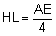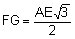< PREVIOUS PROBLEM  |  NEXT PROBLEM >

Problem 50. Triangle with Equilateral triangles. Level: High School, SAT Prep, College

In the figure below, equilateral triangles ABD and BCE are drawn on the sides of a triangle ABC. F, G, and H are the midpoints of AD, CE, and AC respectively. HL and FG are perpendicular. Lines FH, CD, HL,  and FG are cut by line AE at J, O, P, and N respectively. Lines GH, HL, and FG are cut by line CD at K, Q, and M respectively. Prove the following:

1. AE = CD

2. The measure of angle DOE is 120º

3. FH = GH

4. The measure of angle FHG is 120º

5. mÐHFG = mÐHGF = 30º

6. OB is the bisector of ÐDOE

7. mÐOBC = mÐOEC = mÐHGC
mÐADO = mÐABO = mÐAFH

8. OD is the bisector of ÐAOB
OE is the bisector of ÐBOC

9. mÐAOB = mÐBOC = 120º

10. mÐCMG = mÐANF = 30º

11. OB and FG are perpendicular

12. Triangle OPQ is equilateral

13. Triangle JPH is equilateral

14. Triangle HKQ is equilateral

15. HJ = HK + OP

16.17.Hints: See Geometry Help

 Selected Topics

 Home | Geometry | Problems | Open Problems | 10 Problems | 41-50 | Email | By Antonio Gutierrez Post or view a solution to the problem 50